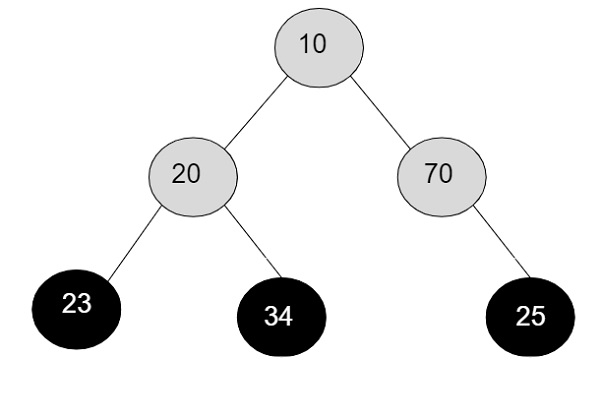# Product of all leaf nodes of binary tree in C++

Given with a binary tree containing nodes and the task is to find the product of all the leaf nodes of a given binary tree.

Leaf nodes are the end nodes which don’t have any children. In a tree, a node can act as a parent node or child node except the root node which can only be a parent node. So the nodes with right and left pointer as NULL are the leaf nodes.

InputOutput

Leaf nodes are -: 23, 34, 25
Product-: 23*34*25 = 19550

## Approach

• Input the node data

• Traverse all the nodes starting from the root node and going to either left sub directory or right subdirectory for traversal.

• Store those nodes with right and left pointer being NULL into a temporary variable to find the product.

• Print the value of a temporary variable holding the multiplied values.

## Algorithm

Start
Step 1 → create structure of a node and temp, next and head as pointer to a
structure node
struct node
int data
Create node *left, *right
End
Step 2 → declare function to insert a node in a tree
node* new_node(int data)
Set node* temp = new node()
Set temp→data = data
Set temp→left = temp→right = NULL
return temp
End
Step 3 → Declare a function to find product of all the leaf nodes
void leaf(node* root, int &product)
IF (!root)
Return
End
IF (!root→left && !root→right)
Set product *= root→data
Call leaf(root→left, product)
Call leaf(root→right, product)
Step 4 → In main()
Create node* root = new_node(10)
Set root→left = new_node(20)
Set root→left→left = new_node(30)
Set int product = 1
Call leaf(root, product)
Display product
Stop

## Example

Live Demo

#include <bits/stdc++.h>
using namespace std;
//structure of a node
struct node{
int data;
node *left, *right;
};
//function to create a new leaf of a tree
node* new_node(int data){
node* temp = new node();
temp→data = data;
temp→left = temp→right = NULL;
return temp;
}
//function to find the product of all leaf nodes of a tree
void leaf(node* root, int &product){
if (!root)
return;
if (!root→left && !root->right)
product *= root→data;
leaf(root→left, product);
leaf(root→right, product);
}
int main(){
node* root = new_node(10);
root→left = new_node(20);
root→left→left = new_node(30);
root→left→right = new_node(40);
root→right = new_node(50);
root→right→right = new_node(60);
root→right→left = new_node(70);
int product = 1;
leaf(root, product);
cout<<"product of a leaf nodes are :"<<product;
return 0;
}

## Output

If run the above code it will generate the following output −

product of a leaf nodes are :5040000